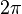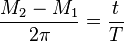# Mean anomaly facts for kids

Kids Encyclopedia Facts

In the study of orbital dynamics the mean anomaly of an orbiting body is the angle the body would have traveled about the center of the orbit's auxiliary circle. Unlike other measures of anomaly, the mean anomaly grows linearly with time. Because of the linear growth with time, the mean anomaly makes calculating the time of flight between two points on the orbit very easy. The mean anomalies for the two points are calculated and their difference is found. Knowing this, the ratio of this difference relative to the entire$2\pi$ encompassing one orbit is simply equal to ratio of the time of flight to the orbital period of one whole orbit (i.e.$\frac{M_2 - M_1}{2\pi} = \frac{t}{T}$).Mean anomaly Facts for Kids. Kiddle Encyclopedia.